﻿ Optimal Design and Performance Analysis of a Grid Connected Photovoltaic System in TogoPublications are Open
Access in this journal
Article Versions
Export Article
• Normal Style
• MLA Style
• APA Style
• Chicago Style
Research Article
Open Access Peer-reviewed

### Optimal Design and Performance Analysis of a Grid Connected Photovoltaic System in Togo

Yendoubé Lare, Koffi Sagna , A. Razak Ali-Tagba
American Journal of Energy Research. 2021, 9(1), 56-74. DOI: 10.12691/ajer-9-1-6
Received June 09, 2021; Revised July 11, 2021; Accepted July 29, 2021

### Abstract

In Togo, the production of electrical energy is mainly hydroelectric and thermal, but the needs remain far greater than the resources; in particular in the city of Lomé, the inhabitants of the city try to fill this energy deficit, among others, by solar systems. Once installed, and in the presence of the sun, solar panels operate continuously until the end of their service, regardless of whether the use is effective or not; which therefore causes losses in the event of overproduction and non-storage. To overcome this problem, we have studied in this article a PV installation (a solar power plant) which is connected to the electrical network, which represents a significant saving in investment and in operation. Thus, in our study, we designed and optimized our PV-Grid model which shows a photovoltaic system connected to the electricity grid through DC / DC boosting power converters and a DC / AC inverter. In this model, we have fixed the meteorological conditions relating to Togo, such as incident solar illumination and temperature, thus making it possible to fix the reference variables Ipvref (photovoltaic current produced) on the DC / DC side and Irmref (final current injected into the network) on the DC / AC side. Thanks to the results obtained, we can recommend this model of photovoltaic system connected to the grid under Togo's conditions and at the same time develop an energy policy and an integration of renewable electricity in Togo strong enough to trigger long-term sustainable development.

### 1. Introduction

The production of energy is a major challenge for the years to come as the world’s energy needs continue to increase. In addition, developing countries will need more and more energy to support their economies. Nowadays, a large part of the global production and consumption of energy causes polluting effects or climatic disturbances. The consumption of natural resources (fossil or fissile) inevitably reduces reserves and compromises their availability for future generations 1.

Today, the global challenge is turned towards renewable energies, in particular solar, wind power, hydraulics, geothermal energy, biomass… Unlike fossil fuels, renewable energies are energies linked to the cycles of the sun and earth. The use of photovoltaic solar energy is the subject of our study 1. We will deal with the problem and technological solutions for connecting a photovoltaic generator to a public distribution grid.

Togo, by virtue of its geographical location, benefits from favourable conditions for the use of renewable energies, in particular solar energy. One of the possibilities of harnessing solar energy is its direct transformation into electrical energy, by means of photovoltaic converters. Several solar projects and programs have been initiated and developed, such as the sizing and management of photovoltaic equipment. Thus the country aims to provide each of the five economic regions with a solar power plant, according to the National Electrification Strategy which aims at access for all Togolese to energy by 2030, against a current rate of nearly 60% 2.

In Togo in particular, large-capacity solar PV projects with injection into the public grid are very few or are being developed and / or implemented (example of the 50 MWp Blitta solar park, etc.) 3. The challenge of these injections into public grids lies not only in their optimized management but above all in their impact in terms of disruption of these grids.

This article aims to optimize a design and simulate a PV plant with minimal loss of energy when connecting the electricity grid. To search for the most suitable equipment for local conditions in order to design, on a given site, a high-performance interconnection-control system from a PV plant to the low-voltage network with optimal injection of active power into the network (public or private).

This article also aims to examine the characteristics of the photovoltaic installations (PV) connected to the national electricity company CEET (Compagnie Energie Electrique du Togo) grid by detailing the stages of dimensioning of the PV installations and then present the optimization algorithm and components in order to optimize these solar system PV.

### 2. Materials and Methods

2.1. Description of the Studied Configuration

The studied configuration consists of a solar PV generator connected to the DC bus of a three-phase voltage inverter, coupled in parallel to the network through an inductor. This electrical network supplies a non-linear receiver consisting of a PD3 rectifier having for load a resistance in series with an inductor 4. The block diagram in figure.1 illustrates this configuration.

2.2. Components of the Photovoltaic System Connected to the Grid

There are several types of connected PV system, the system that we have chosen for our study is shown below and composed of:

- PV Generators;

- DC / DC Converter;

- Inverter and Filter.

• Figure 1. Schematic diagram of a photovoltaic system connected to an electrical network 
2.3. The PV Cell Model

The photovoltaic cell or solar cell is the basic element in the conversion of light energy (solar) into electricity (direct current). A photovoltaic panel consists of several modules, the latter consisting of several cells in series in order to obtain a desired voltage. The power is expressed in Watt peak (Wp), a unit which defines the electrical power available at the terminals of the generator under optimal sunlight conditions of 1000W / m2 at 25°C for Air Mass 1.5 (AM1.5) 5, 6.

The operation of a photovoltaic module is described by the "standard" one-diode model, established by Shokley for a single PV cell 7. It is generalized to a PV module by considering it as a set of identical cells connected in series or in parallel. The PV module of our study system will be made up of the one-diode model, with series and shunt resistors, shown in the following Figure 2:

• Figure 2. Equivalent diagram of a Model PV cell with one diode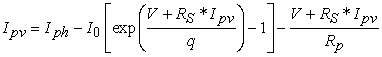(1)

Applying Kirchhoff's law to the circuit, will be obtained by equation (1), which is the current of the cell when we have a series and parallel resistance: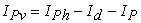(2)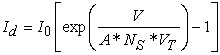(3)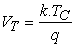(4)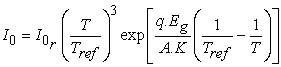(5)

Iph: Photo-current,

Id: Diode current which is proportional to the saturation current and it is given by equation (2) together with equation (4) the saturation current.

VT: Voltage imposed on the diode, it is expressed by equation (3).

I0: Saturation or the leakage current of the diode (A)

I0_r: Inverse current saturation.

VTC: Thermal Voltage, 26mV at 300 K, for silicon cell.

Tc: Actual cell temperature (K).

K: Boltzmann constant (1,38*10-23 J/K)

q: Charge of electrons (1,602*10-19 C).

VT: Thermal stress because of its exclusive use temperature dependence 9.

Ns: Number of PV cells connected in series.

A: Ideality Factor.

After development, we get the global equation of our cell: Equation (5) is the equation that we will use for our simulation of the PV cell. It is not easy to determine the parameters of this transcendental equation. But this model offers the best match with experimental values.

2.4. Principles of Operation for Optimum Operation

In the operation of PV generators (GPVs), for a better efficiency, the technique of the pursuit of the maximum power consists in optimally transferring all the convertible electric power from this source to supply the load. It is essential in all applications using a photovoltaic generator since the criterion of "return on investment" is directly linked to energy production. This is the case for production injected into a grid. The control techniques employed in Maximum Power Point Tracking (MPPT) are 10:

Incremental method applied to the power

This is based on finding the end of the power curve (P-V) obtained directly from the GPV characteristic. The MPP is obtained when the derivative of the power with respect to the voltage vanishes. Among this method, we find:

- The perturbation and observation (P&O) method which will be used in our design and detailed below

- The method by Incrementation of conductance (InC), not detailed 11.

Analog voltage feedback method

This method is based on checking the operation of the panels by comparing the voltage with a reference. This generates an error voltage which varies the PWM (Pulse-Width Modulation) duty cycle of the drive in order to cancel this error 12.

2.5. Method of Perturbation and Observation (P & O)

If you want to follow the maximum power point, you need to know the parameters that modify its position. The use of enslavement techniques using the most flexible, that of perturbation and observation (P&O).

This MPP (Maximum Power Point) tracking algorithm illustrated in Figure 3, is the most used and as its name suggests, it is based on disturbing the system by increasing or decreasing the reference voltage (Vref), or by acting directly on the converter duty cycle. DC / DC, then observe the effect on the output power for possible duty cycle correction.

• Figure 3. Chart of the P&O algorithm
2.6. DC-DC Converter (DC-DC)

DC-DC converters (or Choppers) are used in solar power systems to match the variable amplitude DC source (PV panel) to the load which typically demands a constant DC voltage.

We have three basic conversion circuit topologies (DC-DC):

- Step-up converter (Buck converter)

- Booster converter (Boost converter)

- Step-Up-Booster Converter (Buck-Boost converter)

They are frequently used in photovoltaic systems to generate the desired voltages and currents 13. For our study, the choice is made on the Boost converter, by its ability to boost the current and voltage generated by the GPV.

The characteristics of this type of Boost converter are really very interesting for the design we want to do in Matlab / Simulink.

A Boost converter, or parallel chopper (step-up chopper), is a switching power supply which converts a direct voltage into another direct voltage of higher value. This type of converter can be used as a source-to-load adapter. It is a direct DC – DC converter. The input source is direct current type (inductor in series with a voltage source) and the output load is direct voltage type (capacitor in parallel with the resistive load).

The basic structure and control topology of the booster converter is shown in Figure 4. The equation of state describing the DC-DC step-up converter is given by equation (6) 14.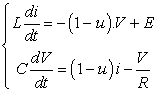(6)
• Figure 4. Electrical circuit diagram of a Boost converter 

Some works 16 have shown that the boost chopper performs better in MPPT applications. In this work, we will focus on an adaptation of a PV system connected to the grid (fixed and with solar tracking) by means of the boost converter.

For our study, we will choose the boost booster chopper in relation to its quality of extracting the maximum energy, which will be an asset for our grid connected system.

2.7. The Inverter Connected to the Grid

The most rewarding photovoltaic applications currently are PV installations intended to supply public electricity grids through inverters that transfer and shape solar electric energy. The progress made in recent years in the development of inverters dedicated to photovoltaics have made it possible to greatly improve these management systems. Inverters are no longer limited to transforming the direct power (DC) generated by the solar panels into alternating power in the form of a sinusoidal voltage of the desired frequency (for example. 230V/400V - 50Hz) but they also exploit the power delivered by the PV generator by forcing it to operate at its maximum power point 17. In addition, they provide reliable grid monitoring to protect the grid against outages and cut off power in the event of problems occurring either in the grid or in the installation. This is absolutely necessary to allow safe intervention on the grid 18.

### 3. Results and Discussion

This section presents a mathematical simulation model that applies to each component of the PV plant connected to the grid. The detailed system (shown in Figure 1) is composed of photovoltaic generator, DC / DC converter, DC / AC inverter controlled by PWM, filter and power grid 1.

3.1. Simulation of the Photovoltaic Generator

After having seen in the previous chapter four how a PV generator is modelled, we performed here the simulation of its operation under Matlab/Simulink.

Figure 5 to Figure 10 below are the representations in Simulink of the various incoming and outgoing currents flowing through the PV generator. The final assembly gives the current Ipv produced by the PV generator under the Matlab / Simulink environment and represented by Figure 11.

• Figure 5. Detailed Iph deployment
• Figure 6. Detailed Id deployment
• Figure 7. Detailed Ish deployment
• Figure 8. Detailed I0 deployment
• Figure 9. Detailed Ipv deployment
• Figure 10. Connection of the different current models
• Figure 11. PV generator diagram in MATLAB-SIMULINK
3.2. Results and Discussions

Simulations, carried out under Matlab / Simulink, show the effect of the variation in illumination and temperature on the I-V and P-V characteristics (Figure 12 and Figure 13). These simulation results prove that the current is directly proportional to the illumination. On the other hand, the voltage does not deteriorate significantly following the decrease in illumination. Indeed, we notice a strong decrease in the short-circuit current with respect to the illumination and a small decrease in the open circuit voltage. This is justified by the fact that the short circuit current is a linear function of the illumination while the open circuit voltage is a logarithmic function. In addition, the voltage is influenced by the variation of the temperature while the short-circuit current varies very little with the temperature. All these results confirm the established knowledge on the characteristics of solar cells.

• Figure 12. Influence of irradiation at T = 25°C
• Figure 13. Influence of temperature at Irr = 1000 Watt/m2
3.3. Simulation of the DC-DC Converter (Overflow Chopper)

For power conversion, it is essential that the efficiency is high to avoid power dissipation and to avoid excessive heating in electronic components. For this reason, all the conversion of exchanged power must be carried out around the energy storage components (inductor and capacitors) and the switches. The power switches used depending on the power level to be converted or controlled. MOSFETs (Metal Oxide Field Effect Transistors) are usually used at relatively low power (a few kW) and IGBTs (Insulated Gate Bipolar Transistors) at higher powers. Thyristors were generally used and accepted at the highest power levels during this simulation.

3.4. Booster CHOPPER SIMulation

Figure 14 represents the simulation of our Chopper Boost chosen, thus the design in Matlab / Simulink is according to the model of Figure 4 of the previous section. To be able to obtain the maximum energy, we have associated with this converter the MPPT P&O control, the cyclic signal of which is shown in Figure 15 below, simulated in Simulink.

• Figure 14. Block diagram of a booster converter
• Figure 15. Functional diagram of a booster converter with detailed block

We can notice in Figure 14 that to this Boost Converter, we have not directly connected our PV module, but rather a DC voltage source, this because we want to analyze the behavior of this Boost Chopper face to any voltage source connected to it.

• Figure 16. DC / DC control signal (trigger- chopper)
• Figure 17. Simulation results of the input voltage of the booster chopper
• Figure 18. Simulation results of the mean value of the output voltage of the booster chopper
• Figure 19. Simulation results of the mean value of the output current of the booster chopper

We control the duty cycle (d) from the value Vpv = Vs using a comparator. We make the comparison with the carrier signal and another sawtooth chosen for the simulation, we get the output signal control shown in Figure 16.

The simulation results of the booster converter are represented in Figure 17 to Figure 19. In Figure 17 the voltage generated by our PV module at the input of the chopper is constant. Figure 18 and Figure 19 respectively represent the output voltage and current of the booster chopper. These results show that the voltage at the booster chopper output is boosted relative to its input value. So, the booster chopper correctly performs its role of optimizing the power supplied by our PV module.

3.5. Simulation of the MPPT P&O Command

Figure 20 illustrates the PWM hysteresis. Below, also the simulation that we made on Matlab of the algorithm of the MPPT method “perturbation-observation” (P&O) where each block represents the different instructions of the figure algorithm Figure 21.

The simulation of the Simulink model of the MPPT (P&O) command block givesthe graph on Figure 22.

The switch control algorithm being shown in the Figure 3, for the opening and closing of the load connection switches at the regulator level, a hysteresis cycle is associated with the result of the Figure 22.

• Figure 20. Simulink block diagram of the PMW control by Hysteresis
• Figure 21. Simulink block diagram of the MPPT P&O control
• Figure 22. MPPT P&O control signal

Figure 22 represents the result obtained after executing the Matlab model preceding the MPPT P&O command. The MPPT control varies the duty cycle of the static converter (SC), using an appropriate electrical signal, to get the maximum power that the GPV (Photovoltaic Generators) can deliver. The MPPT algorithm can be more or less complicated to find the MPP. In general, it is based on the variation of the duty cycle of the CS according to the evolution of the latter's input parameters (I and V and therefore the power of the GPV) until it is placed on the MPP. Several algorithms are available.

It is concluded that the optimization of the power supplied by the GPV (Photovoltaic Generators) is essential in order to have a high efficiency, and the MPPT P&O method allows this optimization.

3.6. Overall System (GPV, Converter, MPPT, Load)

Description of the grid connected system used

Figure 23 illustrates the schematic block of Simulink of the global photovoltaic system adapted by the MPPT command “perturbation and observation”; in this block, we observe the presence of our PV generator with its input parameters (Irradiation, Temperature, Number of cells, Voltage, etc ...).

• Figure 23. Global block diagram of the grid connected PV system

Then we observe the inverter or Boost Chopper which is directly connected to the GPV to which we have connected the MPPT P&O and PWM control described in the previous section in order to boost the values at the output of the component.

Subsequently we associate a DC-AC inverter for the conversion of the direct current produced into alternating current, at the end of which we associate a filter to select the frequencies and amplitudes which are compatible with the electrical network for a good injection with the least possible disturbance.

3.7. Characteristic of the Connected Photovoltaic System

Simulations, carried out under Matlab / Simulink of our solar module of the PV system, show us the effect of the variation of the illumination and of the temperature on the characteristics IV and PV respectively in Figure 24 and Figure 25. These simulation results prove that the current is directly proportional to the illumination. In addition, the voltage is influenced by the variation of the temperature while the short-circuit current varies very little with the temperature.

E = 1000W/m2 et T = 25°C:

• Figure 24. I-V characteristic of a photovoltaic generator
• Figure 25. P-V characteristic of a photovoltaic generator
3.8. Photovoltaic System with MPPT Control (P&O)

The simulation that we performed above concerned the PV system without the MPPT control. Here in the second part of our simulation, we bring into play all the components of our system as well as the grid itself.

PV module

In all the simulations that follow, we have worked with a constant sunshine profile under STC conditions. The control of the booster chopper linking the PV generator to the DC bus by the variation of the duty cycle α allowed us to obtain the following Figure 24 and Figure 25 which illustrate the values of the voltage and current generated by the PV module so therefore at the input of the boost chopper.

Figure 26.a represents the current generated by the photovoltaic generator. According to the characteristics of our PV module. Figure 26.b shows the same current generated on a higher resolution. This signal repeats indefinitely but depending on the conditions imposed on our PV the signal is not the same, and varies especially depending on the irradiation and the temperature.

• Figure 26. Current generated by the photovoltaic generator
• Figure 27. The voltage generated by the photovoltaic generator

Figure 27.a represents the voltage generated by the photovoltaic generator. This tension also follows a given cycle; in the following Figure 27.b enlarges we observe the evolution of this voltage which after reaching a peak, a maximum goes down again. This is due to the components of our system.

Note that this generated voltage was chosen voluntarily by us and may be modified at any time during the simulation; unlike the PV current generated which is linked to irradiation and temperature.

The power generated by the GPV is represented in Figure 28, which is just as repetitive at a given time. This variation is due to the various constraints on the system.

• Figure 28. The power generated by the photovoltaic generator

MPPT method simulation:

The simulation of the MPPT command seen previously was made so that the generator produces its maximum power and allows the voltage to be raised at the level of the DC bus. Thus, we observe in Figure 29 that the MPPT signal follows a cyclic procedure.

Figure 30 and Figure 31 show us how the temporal evolution of the voltage and current at the output of the step-up chopper takes place. We notice that the chopper thus manages to raise the voltage at its input, hence its name elevator or booster.

We observe that at the output, the quantities are increasing; this is due to our MPPT P&O command associated with our BOOST Chopper which is responsible for drawing the maximum current and voltage.

Simulation of the inverter or converter (DC-AC)

As the current supplied by the PV generator to our boost chopper is continuous, to be able to inject into the electrical grid, it will have to be converted into alternating current, hence the need for a DC-AC converter.

Based on simulations of the behavior of electrical quantities generated by the DC-AC inverter, we found the following curves. First, Figure 32 and Figure 35 show us the shape of the current and the voltage generated by the DC-AC inverter at the point of connection to the three-phase power grid. It is well shown that these voltages and currents are perfectly sinusoidal, balanced during their evolution over time.

We also observe a marked increase in the values of the various quantities before the injection into the grid; the signal or the frequency would have to correspond to that of the grid for it to be compatible and for the injection to be able to do with the minimum possible disturbance of the grid. What we know how to ensure during our simulation under Matlab/Simulink.

• Figure 29. DC / DC control signal (trigger- chopper)
• Figure 30. The chopper output voltage of the PV system
• Figure 31. PV system chopper output current
• Figure 32. PV system inverter output current
• Figure 33. The inverter output voltage of the PV

Figure 34 illustrates the different voltages and currents injected into the electrical grid which are sinusoidal and balanced, in order to be in harmony with the grid signal.

Figure 35 represents the same signals but a larger scale. To obtain these results, certain system components intervened, such as the filter, in order to eliminate signals incompatible with the electrical grid.

Figure 36 presents the voltage and current from the overall PV system after conversion and synchronization which will be injected into the network according to its characteristics, to avoid as much as possible disturbances of the entire system connected to the grid.

• Figure 34. Currents injected at the point of connection to the electrical network 3Ф
• Figure 35. Currents injected at the point of connection to the electrical network 3Ф
• Figure 36. The reference voltage, current and synchronous power

### 4. Conclusion

In this work, the grid connected PV system components were implemented in MATLAB / SIMULINK software environments. We have presented the simulation of the grid connected photovoltaic system with the illustration of different operations that the voltage produced by the PV generator undergoes in order to meet the needs of the load. The problem of harmonics was presented, the spectral analysis of the grid current and voltage showed the effect of the harmonic disturbance on the quality of the energy supplied by the GPV and the simulation results showed the efficiency. The addition of a filter at the output of the inverter to improve the quality of the voltage and current injected into the grid has been addressed.

We carried out this study in several stages: the modeling of the photovoltaic system, the static converter, the maximum power point tracking method and the photovoltaic system connected to the electrical network, finally: the simulation of the whole.

In this article, we study among the existing materials for an installation connected to the network which are the components, the most efficient and the best adapted to the region where we receive. Thus, thanks to the different simulations that we have made in this article with the different components of the system connected to the network: at the level of the regulator, the choice of MPPT is more obvious, to which we have associated a hysteresis cycling program which makes it possible to have a better output of electrical energy production and minimal loss throughout the production process. The choice of our inverter, the components of which were detailed in the previous section, gives us an idea of the type of electrical energy supplied to the output which is then diffused and synchronized with the network for a good injection. Also, to minimize losses and at the same time meet the need for consumption, we have opted for a three-phase network.

### References

  Y. BEMANA NGUEOUELE, “Modélisation des injections de puissance d’un système PV sur un réseau public”, Institut International d’Ingénierie de l’Eau et de l’Environnement, 2012. In article  N. M. Ledy, “Le Togo lance les travaux de construction d’une centrale solaire photovoltaïque”, Financial Afrik, févr. 06, 2020. https://www.financialafrik.com/2020/02/06/le-togo-lance-les-travaux-de-construction-dune-centrale-solaire-photovoltaique/ (consulté le juin 08, 2021). In article  T. First, “Les travaux du parc solaire de Blitta de 50 MWc sont achevés”. https://www.togofirst.com/fr/energies/2203-7521-les-travaux-du-parc-solaire-de-blitta-de-50-mwc-sont-acheves (consulté le juin 08, 2021). In article  Boualem BOUKEZATA, Abdelmadjid CHAOUI, Jean Paul GAUBERT, et Mabrouk HACHEMI, “Système solaire photovoltaïque connecté au réseau électrique et associé à un filtre actif parallèle”, Cachan, 2014. In article  SINGO, A.T., “Système d’alimentation photovoltaïque avec stockage hybride pour l’habitat énergétiquement autonome”, Université Henri Poincaré, Nancy-I, 2010. In article  Weidong Xiao, W.G.D., Patrick R. Palmer, et Antoine Capel, “Regulation of Photovoltaic Voltage”, IEEE Transactions on Industrial Electronics, 2007. In article View Article  Z. Benjellouli, “contribution a la modélisation d’une cellule solaire”, Université de Bechar, 2009. In article  Belaout Abdesslam, “Etude et diagnostic des défauts fréquents aux systèmes photovoltaïques (PV) par emploi de la caractéristique courant-tension”, Université de Sétif-1, Alger, Algérie, 2014. In article  Anne, Labouret, Michel, Villoz, Energie photovoltaïque. (Dunod 3eme édition 2006). Bernard Eque, Energie solaire photovoltaïque (Ellipses 2004). Sheik Mohammed, S., 2011. Modeling and simulation of photovoltaic module using MATLAB/Simulink. Int. J. Chem. Environ. Eng. 2. In article  F. Bouchtouche Cherfa, “Etude et réalisation d’une centrale photovoltaïque connectée au réseau de distribution électrique BT”, Mémoire de magister, Ecole National Polytechnique El-Harrach, 2004. In article  G. Adamidis, P.B., et A. Balouktsis, “Photovoltaic system MPPTracker Implementation using a Photovoltaic Array Model with Graphical User Interface”, Institute for Energy Technology; Aalborg University Pontoppidanstraede 101 DK-9220, Aalborg, Denmark. In article  Mabrouk, A., “Etude et conception d’une stratégie de commande d’un onduleur connecté au réseau électrique”, Mémoire de magister en électricité solaire, l’Ecole Nationale Polytechnique El Harrach, Alger, 2008. In article  A. Saadi, “Etude comparative entre les techniques d’optimisation des systèmes de pompage photovoltaïque”, Mémoire de Magister, Université de Biskra, 2000. In article  M. Slama Fateh, “Modélisation D’un Système Multi Générateurs Photovoltaïques Interconnectés Au Réseau Électrique”, Mémoire De Magister, Université Ferhat Abbas – Setif, 2011. In article  M. Zagrouba, M. Bouaïcha, A. Sellami, And M. Ksouric, “Optimisation Par Les Algorithmes Génétiques et Modélisation Par La Méthode SLPV D’un Système Photovoltaïque”, Vème Congrès International Sur Les Energies Renouvelables Et L’environnement, 04-06 Novembre, 2010, Sousse, Tunisie. In article  I. Glasner et J. Appelbaum, “Advantage of boost VS buck topology for maximum power point tracker in photovoltaic systems”, IEEE, 1996. In article  Mme DIDIER et s.l.d.d.M.G., “Les Onduleurs pour Systèmes Photovoltaïques : Fonctionnement”, Hespul, Villeurbanne, août 2007. In article  A. Oi, “Design and simulation of photovoltaic water pumping system”, Thèse de Doctorat, California Polytechnic State University San Luis Obispo, 2005. In article

Published with license by Science and Education Publishing, Copyright © 2021 Yendoubé Lare, Koffi Sagna and A. Razak Ali-TagbaThis work is licensed under a Creative Commons Attribution 4.0 International License. To view a copy of this license, visit http://creativecommons.org/licenses/by/4.0/